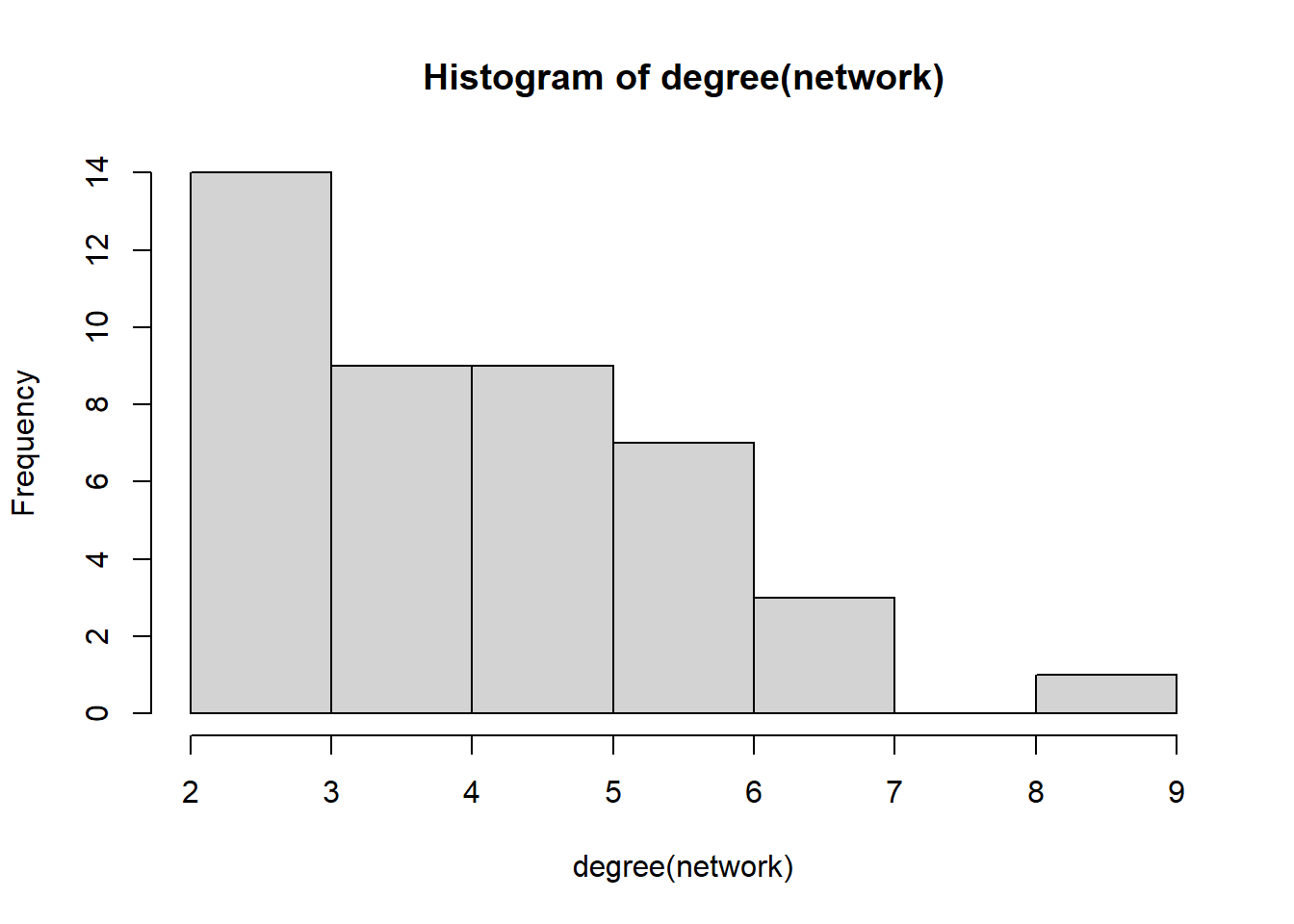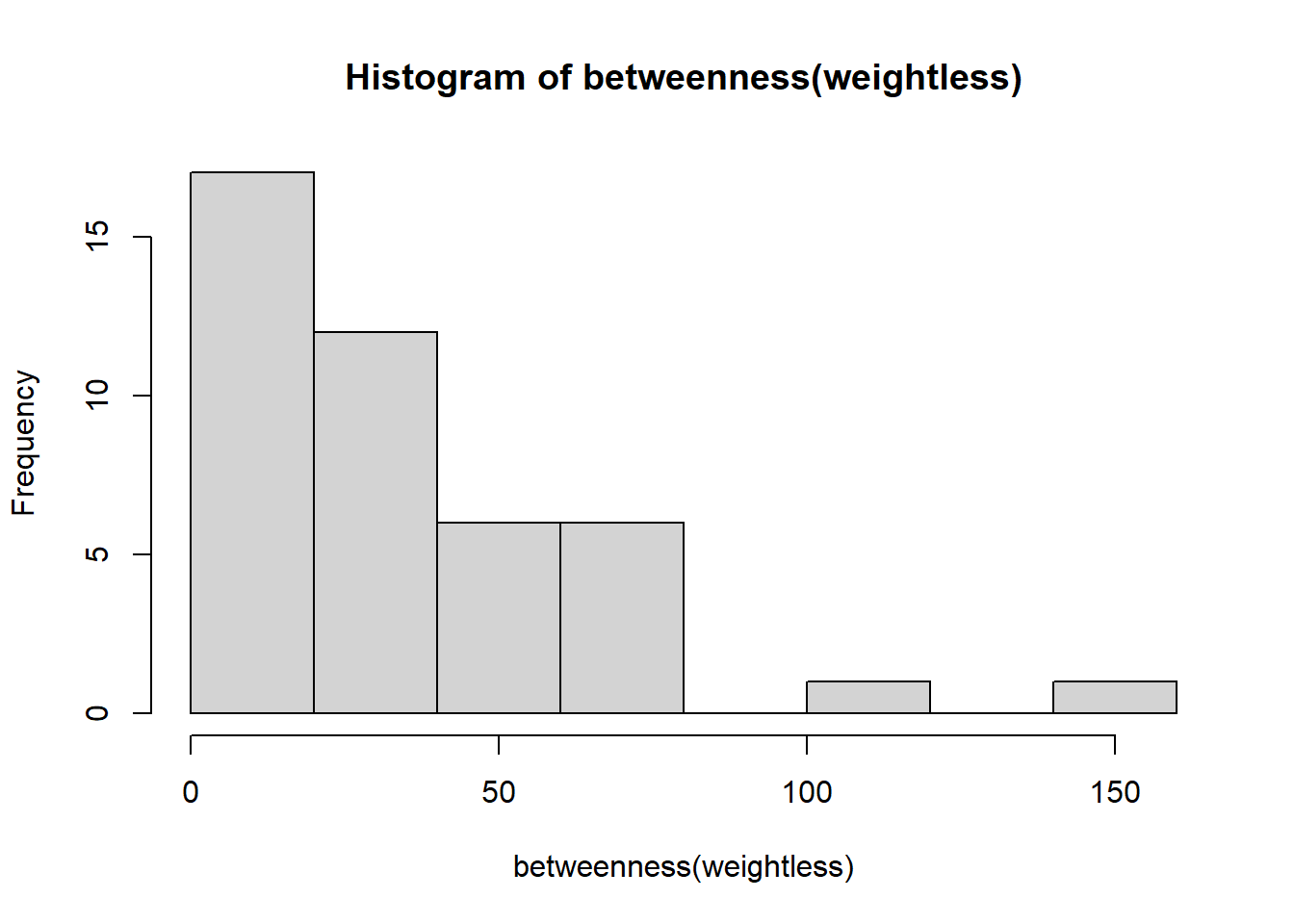## Importing a network

To work with igraph, we first need a network. For this tutorial, we will use the networks from the FlashWeave tutorial: Running FlashWeave on sponge networks).
If you were unable to run FlashWeave, please find the networks here: ZIP file with networks.

library(igraph)
setwd('C:/Users/user/Documents/workshop')
network_loc <- 'C:/Users/user/Documents/workshop/sponges_networks/'  # Change to the folder where you downloaded the files
network = read_graph(paste(network_loc, 'Axinellida.gml', sep=''), format='gml')
##
## Attaching package: 'igraph'
## The following objects are masked from 'package:stats':
##
##     decompose, spectrum
## The following object is masked from 'package:base':
##
##     union

## Accessing the igraph object

What information is already in the network? We can see the node and edge metadata by accessing a single node or edge.

print(V(network))
print(E(network))

vertex_attributes <- get.vertex.attribute(network)
edge_attributes <- get.edge.attribute(network)

print(vertex_attributes)
print(edge_attributes) 

Unfortunately, the taxonomy is not contained in the node metadata. This we can address by reading the original BIOM file. You can download the processed BIOM files from the Github repository: link to zip BIOM files Unzip the files in a location of your choice.
We can read the BIOM file with phyloseq, extract the taxonomic information and add this to the networks.

The command below adds the taxonomy for the Axinellida network; can you print the complete taxonomy for taxon c4357330?

library(phyloseq)
biom_loc <- ('C:/Users/user/Documents/workshop/sponges/')  # Change to the folder where you downloaded the files
biom_file <- import_biom(paste(biom_loc, "Axinellida.biom", sep=''))
tax <- data.frame(tax_table(biom_file))

for (col in colnames(tax)){
network <- set.vertex.attribute(network, value=tax[[col]], name=col)
}

Of course, we can repeat this for all sponge networks. First, we make a list of all names from the folders containing the networks. Then we find the matching BIOM files. Finally, we store these networks as graphml files instead, so they can be imported in other software. For example, you can load these files into Cytoscape to visualize the networks. We also store them in a named list so they can be used for other analyses.

# Get a list of all files with glm extension
network_names <- list.files("sponges_networks", pattern = "\\.gml$") network_list <- list() for (name in network_names){ clean_name <- substr(name, 1, nchar(name)-4) network = read_graph(paste(network_loc, name, sep=''), format='gml') # read BIOM file biom_file <- import_biom(paste(biom_loc, clean_name, '.biom', sep='')) tax <- data.frame(tax_table(biom_file)) for (col in colnames(tax)){ network <- set.vertex.attribute(network, value=tax[[col]], name=col) network_list[[clean_name]] <- network name <- paste(clean_name, '.graphml', sep='') #write_graph(network, file=name, format="graphml") uncomment to write graphml file } } If we need to calculate network properties for networks, we can do so via igraph. For example, the following code calculates the degree, betweenness centrality, degree assortativity and generates a shortest path in the Axinellida network. We need to remove edge weights for 2 of these calculations. network <- network_list[['Axinellida']] hist(degree(network))print(paste("Degree assortativity:", assortativity.degree(network))) ##  "Degree assortativity: -0.0476190476190478" weightless <- delete_edge_attr(network, "weight") hist(betweenness(weightless))shortest_paths(weightless, 1, 2)$vpath
## []
## + 3/43 vertices, from e2fe2cf:
##   1 39  2

For an overview of the functions in igraph, check out the reference manual.

Back to overview